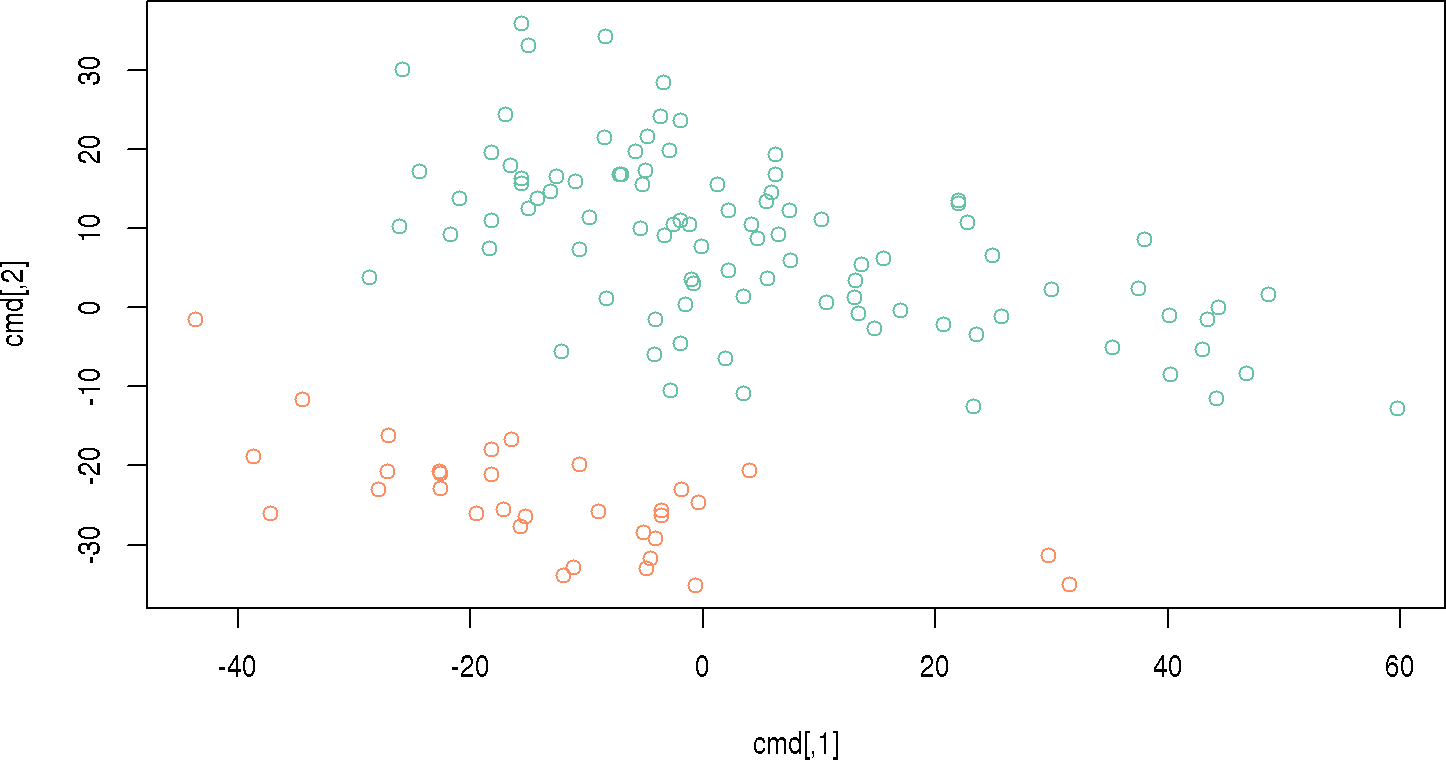# 1 Exploration and simple univariate measures

path <- file.choose()    # look for BRFSS-subset.csv
stopifnot(file.exists(path))
brfss <- read.csv(path)

## 1.1 Clean data

R read Year as an integer value, but it’s really a factor

brfss$Year <- factor(brfss$Year)

## 1.2 Weight in 1990 vs. 2010 Females

Create a subset of the data

brfssFemale <- brfss[brfss$Sex == "Female",] summary(brfssFemale) ## Age Weight Sex Height Year ## Min. :18.00 Min. : 36.29 Female:12039 Min. :105.0 1990:5718 ## 1st Qu.:37.00 1st Qu.: 57.61 Male : 0 1st Qu.:157.5 2010:6321 ## Median :52.00 Median : 65.77 Median :163.0 ## Mean :51.92 Mean : 69.05 Mean :163.3 ## 3rd Qu.:67.00 3rd Qu.: 77.11 3rd Qu.:168.0 ## Max. :99.00 Max. :272.16 Max. :200.7 ## NA's :103 NA's :560 NA's :140 Visualize plot(Weight ~ Year, brfssFemale)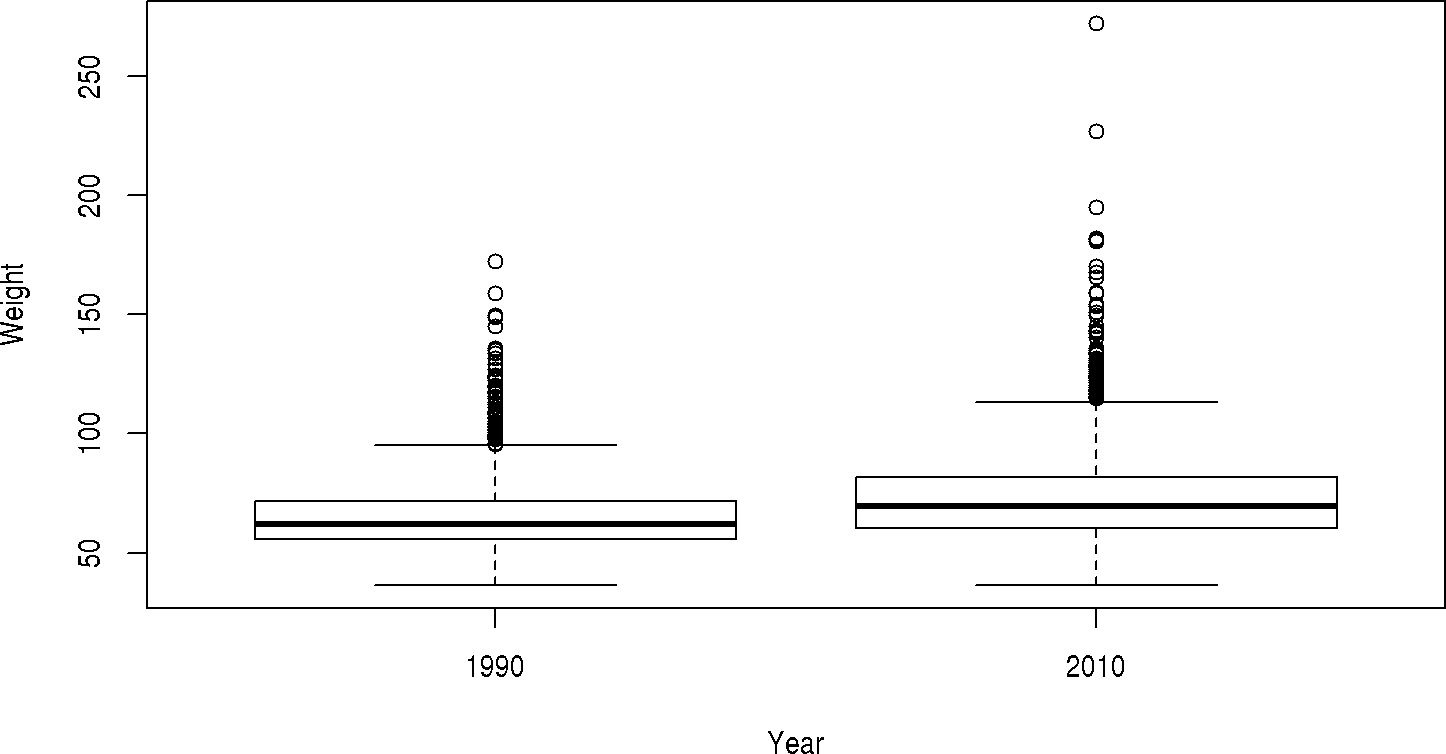Statistical test t.test(Weight ~ Year, brfssFemale) ## ## Welch Two Sample t-test ## ## data: Weight by Year ## t = -27.133, df = 11079, p-value < 2.2e-16 ## alternative hypothesis: true difference in means is not equal to 0 ## 95 percent confidence interval: ## -8.723607 -7.548102 ## sample estimates: ## mean in group 1990 mean in group 2010 ## 64.81838 72.95424 ## 1.3 Weight and height in 2010 Males Create a subset of the data brfss2010Male <- subset(brfss, Year == 2010 & Sex == "Male") summary(brfss2010Male) ## Age Weight Sex Height Year ## Min. :18.00 Min. : 36.29 Female: 0 Min. :135 1990: 0 ## 1st Qu.:45.00 1st Qu.: 77.11 Male :3679 1st Qu.:173 2010:3679 ## Median :57.00 Median : 86.18 Median :178 ## Mean :56.25 Mean : 88.85 Mean :178 ## 3rd Qu.:68.00 3rd Qu.: 99.79 3rd Qu.:183 ## Max. :99.00 Max. :278.96 Max. :218 ## NA's :30 NA's :49 NA's :31 Visualize the relationship hist(brfss2010Male$Weight)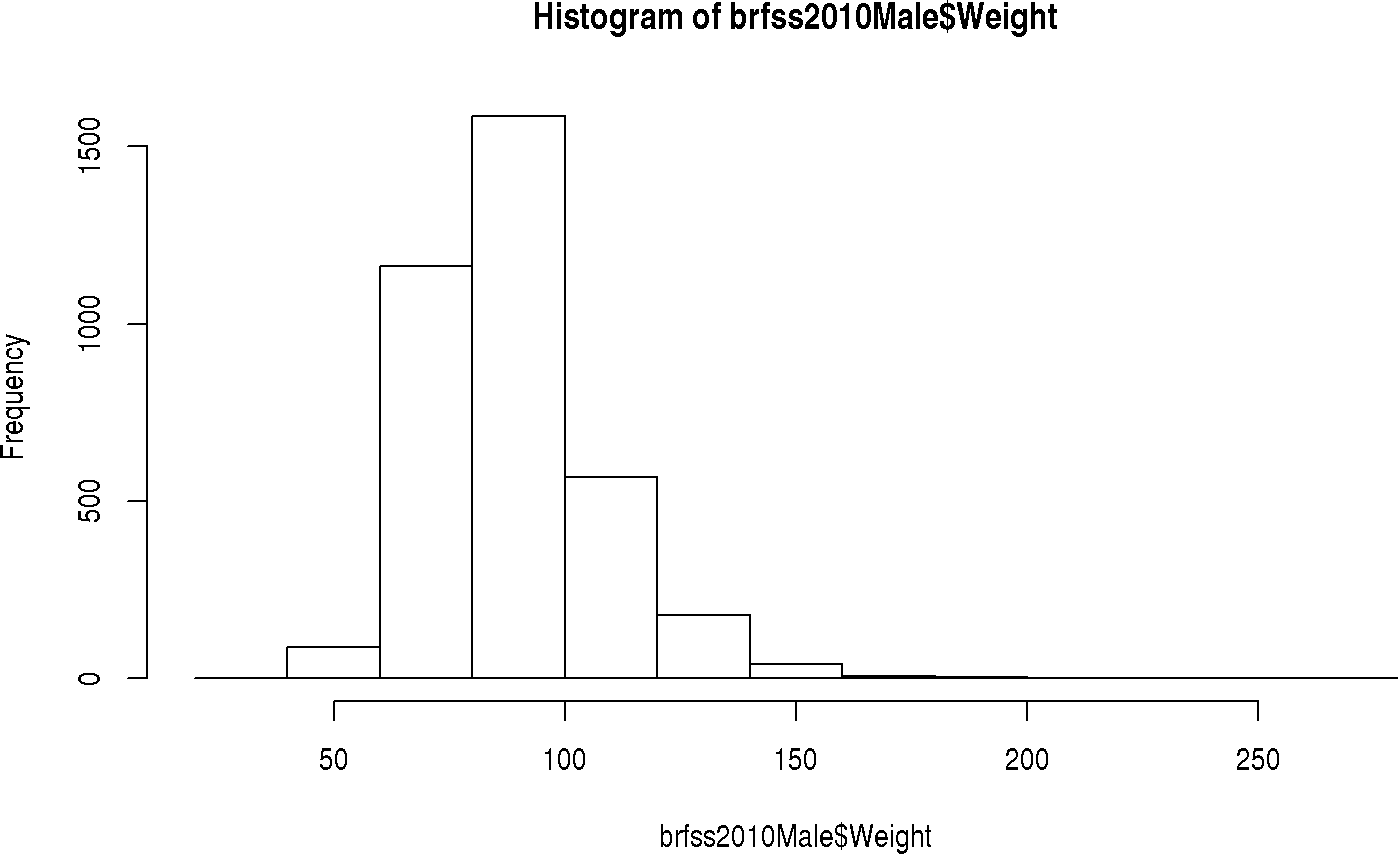hist(brfss2010Male$Height)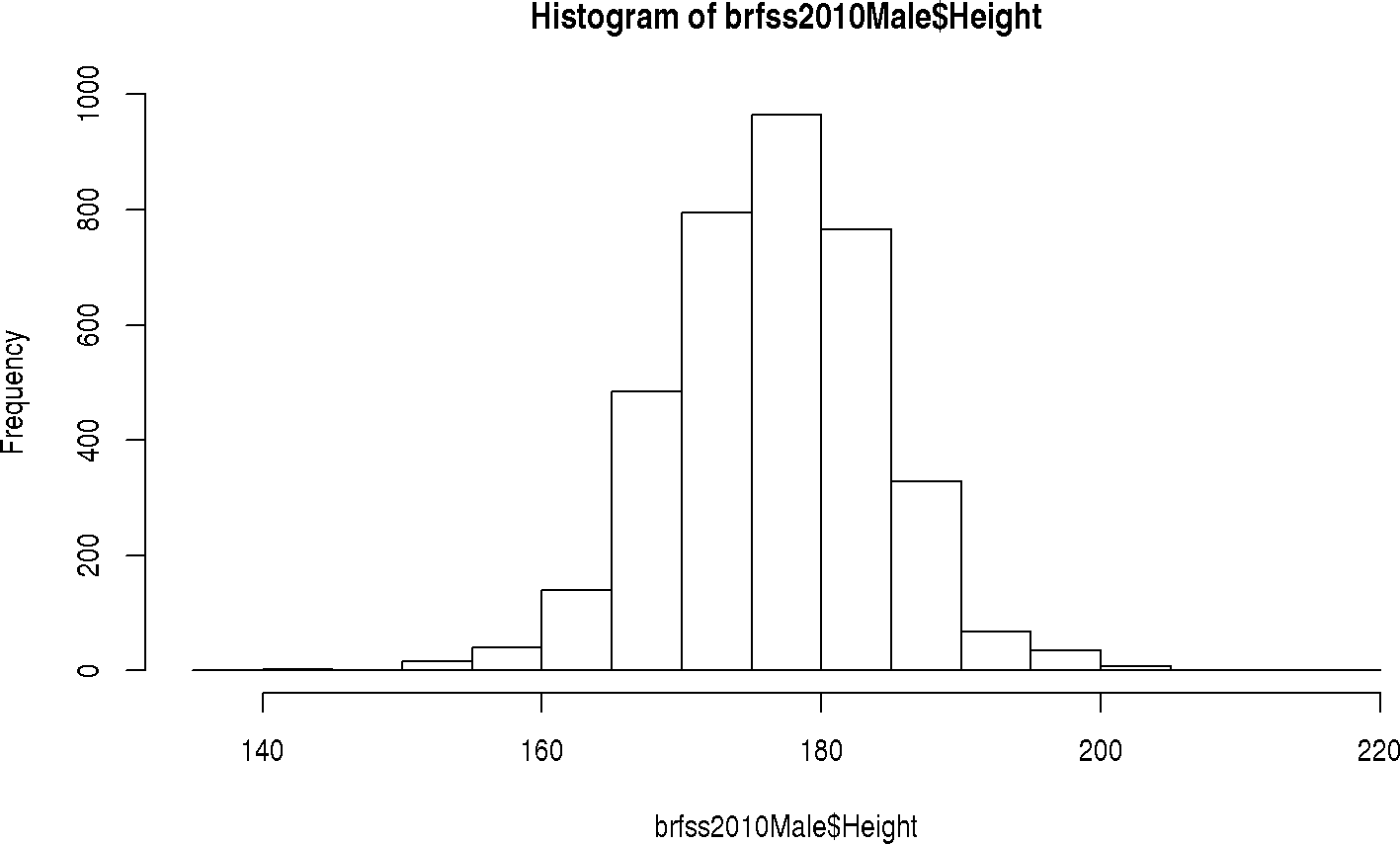plot(Weight ~ Height, brfss2010Male)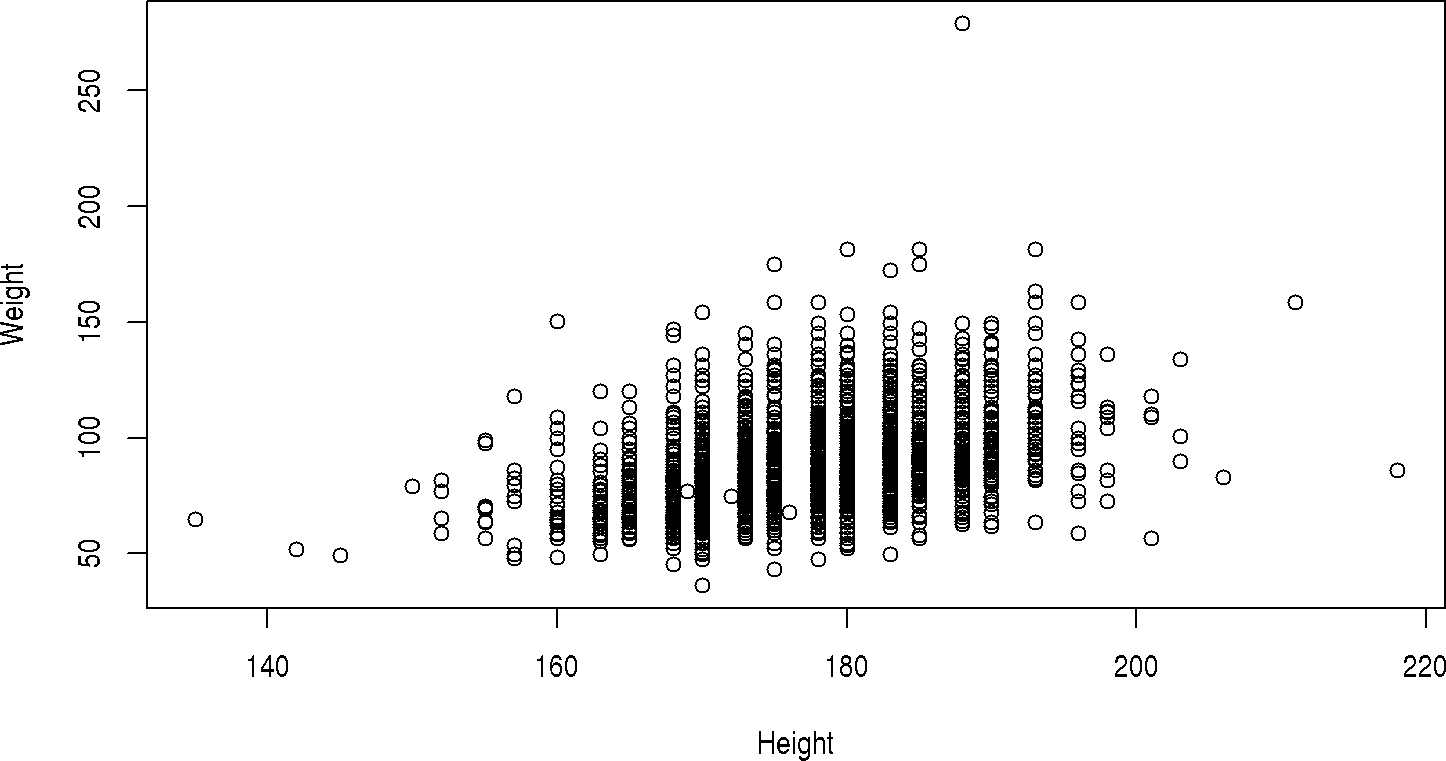Fit a linear model (regression) fit <- lm(Weight ~ Height, brfss2010Male) fit ## ## Call: ## lm(formula = Weight ~ Height, data = brfss2010Male) ## ## Coefficients: ## (Intercept) Height ## -86.8747 0.9873 Summarize as ANOVA table anova(fit) ## Analysis of Variance Table ## ## Response: Weight ## Df Sum Sq Mean Sq F value Pr(>F) ## Height 1 197664 197664 693.8 < 2.2e-16 *** ## Residuals 3617 1030484 285 ## --- ## Signif. codes: 0 '***' 0.001 '**' 0.01 '*' 0.05 '.' 0.1 ' ' 1 Plot points, superpose fitted regression line; where am I? plot(Weight ~ Height, brfss2010Male) abline(fit, col="blue", lwd=2) points(180, 88, col="red", cex=4, pch=20)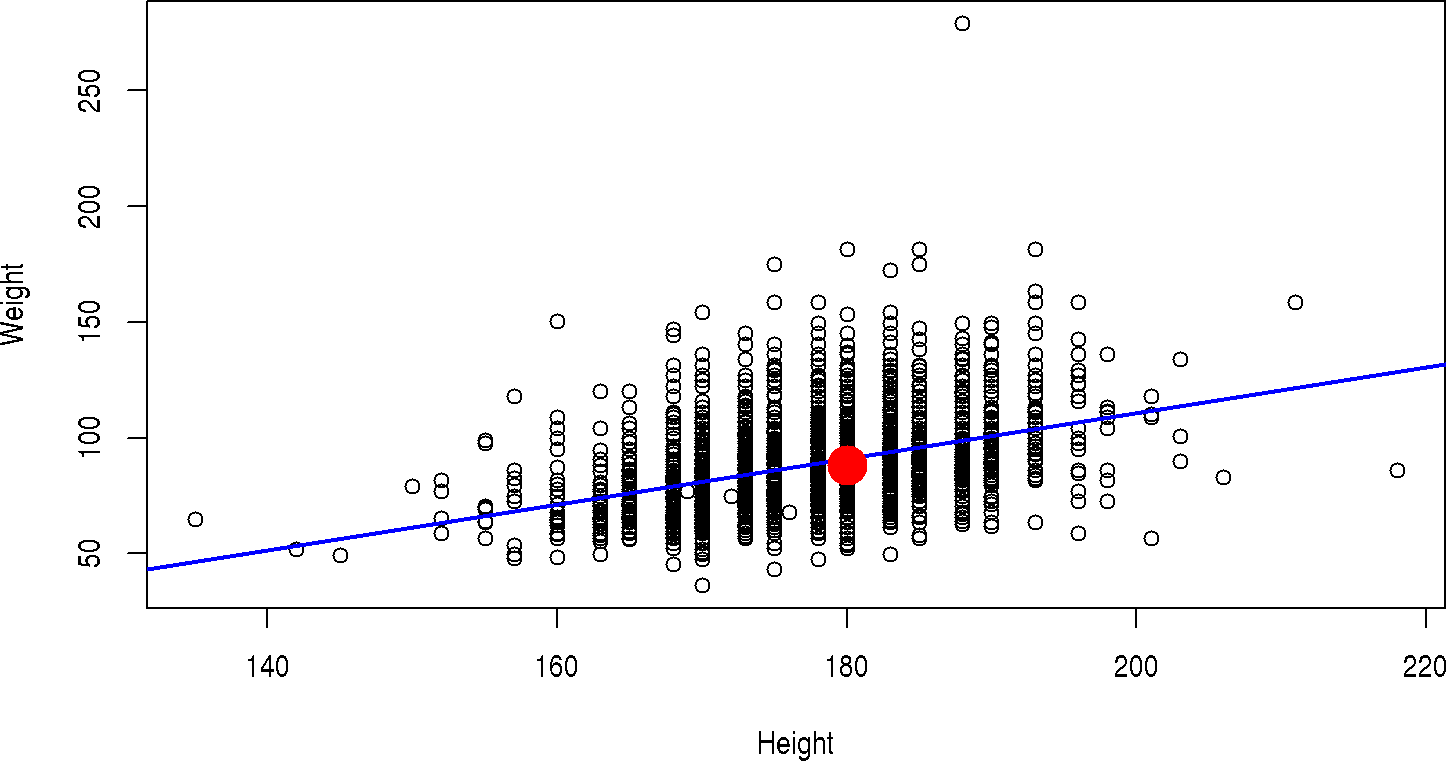Class and available ‘methods’ class(fit) # 'noun' methods(class=class(fit)) # 'verb' Diagnostics plot(fit) ?plot.lm # 2 Multivariate analysis This is a classic microarray experiment. Microarrays consist of ‘probesets’ that interogate genes for their level of expression. In the experiment we’re looking at, there are 12625 probesets measured on each of the 128 samples. The raw expression levels estimated by microarray assays require considerable pre-processing, the data we’ll work with has been pre-processed. ## 2.1 Input and setup Start by finding the expression data file on disk. path <- file.choose() # look for ALL-expression.csv stopifnot(file.exists(path)) The data is stored in ‘comma-separate value’ format, with each probeset occupying a line, and the expression value for each sample in that probeset separated by a comma. Input the data using read.csv(). There are three challenges: 1. The row names are present in the first column of the data. Tell R this by adding the argument row.names=1 to read.csv(). 2. By default, R checks that column names do not look like numbers, but our column names do look like numbers. Use the argument check.colnames=FALSE to over-ride R’s default. 3. read.csv() returns a data.frame. We could use a data.frame to work with our data, but really it is a matrix() – the columns are of the same type and measure the same thing. Use as.matrix() to coerce the data.frame we input to a matrix. exprs <- read.csv(path, row.names=1, check.names=FALSE) exprs <- as.matrix(exprs) class(exprs) ##  "matrix" dim(exprs) ##  12625 128 exprs[1:6, 1:10] ## 01005 01010 03002 04006 04007 04008 04010 ## 1000_at 7.597323 7.479445 7.567593 7.384684 7.905312 7.065914 7.474537 ## 1001_at 5.046194 4.932537 4.799294 4.922627 4.844565 5.147762 5.122518 ## 1002_f_at 3.900466 4.208155 3.886169 4.206798 3.416923 3.945869 4.150506 ## 1003_s_at 5.903856 6.169024 5.860459 6.116890 5.687997 6.208061 6.292713 ## 1004_at 5.925260 5.912780 5.893209 6.170245 5.615210 5.923487 6.046607 ## 1005_at 8.570990 10.428299 9.616713 9.937155 9.983809 10.063484 10.662059 ## 04016 06002 08001 ## 1000_at 7.536119 7.183331 7.735545 ## 1001_at 5.016132 5.288943 4.633217 ## 1002_f_at 3.576360 3.900935 3.630190 ## 1003_s_at 5.665991 5.842326 5.875375 ## 1004_at 5.738218 5.994515 5.748350 ## 1005_at 11.269115 8.812869 10.165159 range(exprs) ##  1.984919 14.126571 We’ll make use of the data describing the samples path <- file.choose() # look for ALL-phenoData.csv stopifnot(file.exists(path)) pdata <- read.csv(path, row.names=1) class(pdata) ##  "data.frame" dim(pdata) ##  128 21 head(pdata) ## cod diagnosis sex age BT remission CR date.cr t.4.11. t.9.22. ## 01005 1005 5/21/1997 M 53 B2 CR CR 8/6/1997 FALSE TRUE ## 01010 1010 3/29/2000 M 19 B2 CR CR 6/27/2000 FALSE FALSE ## 03002 3002 6/24/1998 F 52 B4 CR CR 8/17/1998 NA NA ## 04006 4006 7/17/1997 M 38 B1 CR CR 9/8/1997 TRUE FALSE ## 04007 4007 7/22/1997 M 57 B2 CR CR 9/17/1997 FALSE FALSE ## 04008 4008 7/30/1997 M 17 B1 CR CR 9/27/1997 FALSE FALSE ## cyto.normal citog mol.biol fusion.protein mdr kinet ccr ## 01005 FALSE t(9;22) BCR/ABL p210 NEG dyploid FALSE ## 01010 FALSE simple alt. NEG <NA> POS dyploid FALSE ## 03002 NA <NA> BCR/ABL p190 NEG dyploid FALSE ## 04006 FALSE t(4;11) ALL1/AF4 <NA> NEG dyploid FALSE ## 04007 FALSE del(6q) NEG <NA> NEG dyploid FALSE ## 04008 FALSE complex alt. NEG <NA> NEG hyperd. FALSE ## relapse transplant f.u date.last.seen ## 01005 FALSE TRUE BMT / DEATH IN CR <NA> ## 01010 TRUE FALSE REL 8/28/2000 ## 03002 TRUE FALSE REL 10/15/1999 ## 04006 TRUE FALSE REL 1/23/1998 ## 04007 TRUE FALSE REL 11/4/1997 ## 04008 TRUE FALSE REL 12/15/1997 Some of the results below involve plots, and it’s convenient to choose pretty and functional colors. We use the RColorBrewer package; see colorbrewer.org library(RColorBrewer) ## not available? install package via RStudio highlight <- brewer.pal(3, "Set2")[1:2] highlight’ is a vector of length 2, light and dark green. For more options see ?RColorBrewer and to view the predefined palettes display.brewer.all() ## 2.2 Cleaning We’ll add a column to pdata, derived from the BT column, to indicate whether the sample is B-cell or T-cell ALL. pdata$BorT <- factor(substr(pdata$BT, 1, 1)) Microarray expression data is usually represented as a matrix of genes as rows and samples as columns. Statisticians usually think of their data as samples as rows, features as columns. So we’ll transpose the expression values exprs <- t(exprs) Confirm that the pdata rows correspond to the exprs rows. stopifnot(identical(rownames(pdata), rownames(exprs))) ## 2.3 Unsupervised machine learning – multi-dimensional scaling Reduce high-dimensional data to lower dimension for visualization. Calculate distance between samples (requires that the expression matrix be transposed). d <- dist(exprs) Use the cmdscale() function to summarize the distance matrix into two points in two dimensions. cmd <- cmdscale(d) Visualize the result, coloring points by B- or T-cell status plot(cmd, col=highlight[pdata$BorT])`